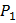# A thin prismwith anglemade from a glass of refractive index 1.54 is combined with another thin prismmade from glass of refractive index 1.72 to produce dispersion without deviation. The angle of the prismis a)b)c)d)## Question ID - 152339 :- A thin prismwith anglemade from a glass of refractive index 1.54 is combined with another thin prismmade from glass of refractive index 1.72 to produce dispersion without deviation. The angle of the prismis a)b)c)d)3537

 (c) For no deviation, (Negative sign implies that two prisms should be connected in opposition.
 The plane face of a planoconvex lens is silvered. Ifbe the refractive index andthe radius of curvature of curved surface, then the system will behave like a concave mirror of radius of curvature a)b)c)d)